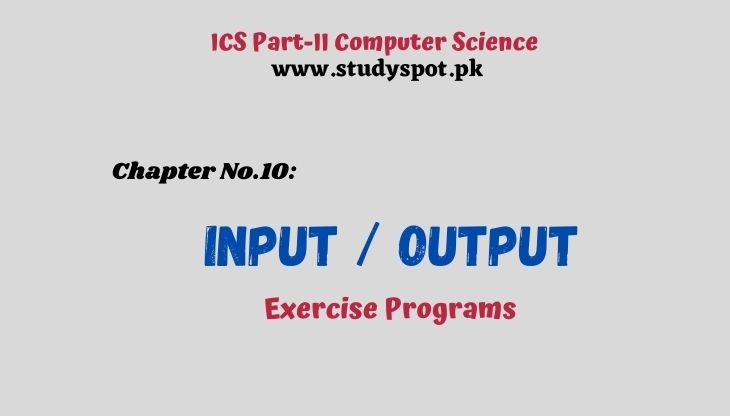# Input Output C ProgramsInput Output C Programs. This post is for ICS Part-II | 2nd Year Computer Science Chapter No.10. Input/Output. The following programs along with answers are important for the final exams.

We have tried our best to provide the best solution for exercise programs, so stay tuned for more C programs | short questions and answers | MCQs | Online Test/Quiz.

### Chapter No.10: Input/Output

#### Exercise Programs in C

Q.8(a): Show how the value -17.246 would be printed using the formats %8.4f, %8.3f, %8.2f, %8.1f, %8.0f, and %0.2f.

%8.4f                     -17.2460

%8.3f                     □-17.246

%8.2f                     □□-17.25

%8.1f                     □□□-17.2

%8.0f                     □□□□□-17

%0.2f                     □□-17.25

The following figure shows how the value -17.246 would be printed using the formats %8.4f, %8.3f, %8.2f, %8.1f, %8.0f, and %0.2f.Q.8(b): Assuming x (type double) is 21.335 and y (type int) is 200, show the output of the following statements (on paper). For clarity, use the symbol □ shows a blank space).

printf("x is %6.2f \t y is %4d\n",x,y);

Output: x is 21.34              y is 200

printf("y is %d\n",y);

Output: y is 200

printf("x is %.1f\n",x);

Output: x is 21.3

The following figure shows the output screen of the above-mentioned statements:

Q.8(c): If the variables a, b, and c are 307, 408.558, and -12.31, respectively, write a statement that will display the following line: (for clarity, the symbol □ shows a blank space).

□□307□□□□□408.56□□□□-12.3

Following is the code that will display the above line/output:

printf("%5d%11.2f%9.1f",a,b,c);

Q.9: Write a program that asks the user to enter the radius of a circle and then computes and displays the circle’s area. Use the formula.

#include<stdio.h>

#include<math.h>

#define PI 3.14159

void main()

{

printf("Area of Circle is =%.2f",area);

getch();

}

Q.10: Write a program that stores the values ‘A’, ‘U’, 3.456E10, and 50 in separate memory cells. Your program should get the first three values as input data but use an assignment statement to store the last value.

#include<stdio.h>

#include<conio.h>

main()

{

char ch1,ch2;

float a;

int b;

// INPUT STATEMENTS

printf("Enter 1st Character:");

ch1=getche();

printf("\nEnter 2nd Character:");

ch2=getche();

printf("\nEnter Exponential Value:");

scanf("%e",&a);  // 3rd Value

b=50;  // 4th Value

// OUTPUT STATEMENTS

printf("\n\n====OUTPUT=====");

printf("\n\n 1st Character:%c",ch1);

printf("\n2nd Character:%c",ch2);

printf("\n1st Value:%e",a);

printf("\n2nd Value:%d\n\n",b);

getch();

}

Q.11: Write a that converts a temperature in degrees Fahrenheit to degrees Celsius. For conversion, use the following formula.

celsius = 5/9 (Fahrenheit – 32)

#include<stdio.h>

#include<conio.h>

void main()

{

float celsius,fahrenheit;

printf("Enter the temperature in Fahrenheit=");

scanf("%f",&fahrenheit);

celsius=5.0/9.0*(fahrenheit-32.0);

printf("Temperature in Celsius = %.2f",celsius);

getch();

}

Q.12: Write a program that takes a positive number with a fractional part and rounds it to two decimal places. For example, 25.4851 would round to 25.49, and 62.4431 would round to 62.44.

#include<stdio.h>

#include<conio.h>

main()

{

float a;

printf("Enter any number:");

scanf("%f",&a);

printf("\nNumber with two decimal places:%.2f",a);

getch();

}